• 向量自回归模型简称VAR模型，是一种常用计量经济模型，1980年由克里斯托弗·西姆斯(Christopher Sims)提出。VAR模型是用模型中所有当期变量对所有变量若干滞后变量进行回归。VAR模型用来估计联合内生变量的动态...
向量自回归模型简称VAR模型，是一种常用的计量经济模型，1980年由克里斯托弗·西姆斯(Christopher Sims)提出。VAR模型是用模型中所有当期变量对所有变量的若干滞后变量进行回归。VAR模型用来估计联合内生变量的动态关系，而不带有任何事先约束条件。它是AR模型的推广，此模型目前已得到广泛应用。 向量自回归(VAR)是基于数据的统计性质建立模型，VAR模型把系统中每一个内生变量作为系统中所有内生变量的滞后值的函数来构造模型，从而将单变量自回归模型推广到由多元时间序列变量组成的“向量”自回归模型。VAR模型是处理多个相关经济指标的分析与预测最容易操作的模型之一，并且在一定的条件下，多元MA和ARMA模型也可转化成VAR模型，因此近年来VAR模型受到越来越多的经济工作者的重视。image.png利用 2005 年 8 月汇率改革后消费者价格指数(CPI)和人民币名义有效汇率(NEER)的月度数据建立两变量 VAR 模型来分析人民币汇率变动与CPI之间的动态关系。实验准备> data > data > data > colnames(data) > data > data > CPI > NEER > length(CPI) 137> length(NEER) 137可以看到数据长度为 137，即包含了 137 个月度 CPI 和 NEER 数据。下面绘制两个变量的时序图，观察消费者价格指数和名义汇率的波动情况。> CPI.ts > NEER.ts > par(mfrow=c(2,1))> plot(CPI.ts, type="l", xlab="Date", ylab="CPI")> plot(NEER.ts, type="l", xlab="Date", ylab="NEER")image.png可以看到消费者价格指数(CPI)的波动幅度较大，而名义有效汇率波动较小但明显呈现一个波动上升的趋势，两个变量序列大致是不平稳的。平稳性检验在拟合 VAR 模型之前，需要对变量进行平稳性检验，如果要拟合的内生变量都是平稳的或者同阶单整的才可进行 VAR 模型的拟合。但在平稳性检验之前需要先项两变量取对数，以消除时间序列的异方差的影响。> lncpi > lnneer 进行平稳性检验本实验选择 urca 包中的 ur.df 函数进行单位根检验，若存在单位根则序列不平稳；反之，不存在单位根则序列平稳。> library(urca)> urt.lncpi > urt.lnneer > summary(urt.lncpi)################################################ Augmented Dickey-Fuller Test Unit Root Test ################################################Test regression trendCall:lm(formula = z.diff ~ z.lag.1 + 1 + tt + z.diff.lag)Residuals:Min        1Q    Median        3Q       Max-0.028676 -0.003311  0.000381  0.003035  0.043118Coefficients:Estimate Std. Error t value Pr(>|t|)(Intercept)  3.047e-01  1.477e-01   2.063   0.0411 *z.lag.1     -6.555e-02  3.185e-02  -2.058   0.0416 *tt          -1.443e-05  1.678e-05  -0.860   0.3912z.diff.lag  -1.178e-01  8.627e-02  -1.365   0.1745---Signif. codes:0 ‘***’ 0.001 ‘**’ 0.01 ‘*’ 0.05 ‘.’ 0.1 ‘ ’ 1Residual standard error: 0.007505 on 131 degrees of freedomMultiple R-squared:  0.05455,   Adjusted R-squared:  0.0329F-statistic: 2.519 on 3 and 131 DF,  p-value: 0.06084Value of test-statistic is: -2.0578 1.5223 2.2719Critical values for test statistics:1pct  5pct 10pcttau3 -3.99 -3.43 -3.13phi2  6.22  4.75  4.07phi3  8.43  6.49  5.47> summary(urt.lnneer)################################################ Augmented Dickey-Fuller Test Unit Root Test ################################################Test regression trendCall:lm(formula = z.diff ~ z.lag.1 + 1 + tt + z.diff.lag)Residuals:Min        1Q    Median        3Q       Max-0.038574 -0.007164 -0.000990  0.009182  0.033482Coefficients:Estimate Std. Error t value Pr(>|t|)(Intercept)  0.4429099  0.1380821   3.208  0.00168 **z.lag.1     -0.0995380  0.0312008  -3.190  0.00178 **tt           0.0003157  0.0001058   2.983  0.00341 **z.diff.lag   0.4099249  0.0810914   5.055 1.42e-06 ***---Signif. codes:0 ‘***’ 0.001 ‘**’ 0.01 ‘*’ 0.05 ‘.’ 0.1 ‘ ’ 1Residual standard error: 0.01267 on 131 degrees of freedomMultiple R-squared:  0.1877,    Adjusted R-squared:  0.1691F-statistic: 10.09 on 3 and 131 DF,  p-value: 5.01e-06Value of test-statistic is: -3.1902 4.2783 5.1509Critical values for test statistics:1pct  5pct 10pcttau3 -3.99 -3.43 -3.13phi2  6.22  4.75  4.07phi3  8.43  6.49  5.47判断是否平稳主要看详细拟合结果的最后两个部分，即：1.Value of test-statistic is:2.Critical values for test statistics:1 是检验统计量的值，2 是对应的显著性水平下检验统计量的临界值。 单位根检验的原假设是序列存在单位根。lncpi的检验统计量的值 -2.0578 在 1%、5%、10% 的显著性水平下都大于临界值(-3.99 -3.43 -3.13)，则不能拒绝原价设而接受存在单位根的假设，说明 lncpi 序列是不平稳的。(注意，统计量的值和临界值为负，统计量的值大于临界值是接受原假设；若统计量的值和临界值为正值，统计量的值大于临界值是拒绝原假设。)同理，lnneer 的检验统计量的值 -3.1902 在 1% 、5% 的显著性水平下都大于对应的临界值(-3.99 -3.43 -3.13)，不能拒绝原假设，lnneer 序列也是不平稳的，存在单位根。由于两个变量都存在单位根，对序列差分后检验序列的平稳性。> dlncpi > dlnneer > urt.dlncpi > urt.dlnneer > summary(urt.dlncpi)################################################ Augmented Dickey-Fuller Test Unit Root Test ################################################Test regression trendCall:lm(formula = z.diff ~ z.lag.1 + 1 + tt + z.diff.lag)Residuals:Min        1Q    Median        3Q       Max-0.029902 -0.003086  0.000184  0.003073  0.043732Coefficients:Estimate Std. Error t value Pr(>|t|)(Intercept)  6.399e-04  1.345e-03   0.476    0.635z.lag.1     -1.086e+00  1.327e-01  -8.189 2.13e-13 ***tt          -8.213e-06  1.710e-05  -0.480    0.632z.diff.lag  -5.347e-02  8.749e-02  -0.611    0.542---Signif. codes:0 ‘***’ 0.001 ‘**’ 0.01 ‘*’ 0.05 ‘.’ 0.1 ‘ ’ 1Residual standard error: 0.007642 on 130 degrees of freedomMultiple R-squared:  0.5753,    Adjusted R-squared:  0.5655F-statistic:  58.7 on 3 and 130 DF,  p-value: < 2.2e-16Value of test-statistic is: -8.1895 22.3559 33.5337Critical values for test statistics:1pct  5pct 10pcttau3 -3.99 -3.43 -3.13phi2  6.22  4.75  4.07phi3  8.43  6.49  5.47> summary(urt.dlnneer)################################################ Augmented Dickey-Fuller Test Unit Root Test ################################################Test regression trendCall:lm(formula = z.diff ~ z.lag.1 + 1 + tt + z.diff.lag)Residuals:Min        1Q    Median        3Q       Max-0.038709 -0.008143  0.000447  0.008358  0.032081Coefficients:Estimate Std. Error t value Pr(>|t|)(Intercept)  2.369e-03  2.342e-03   1.011    0.314z.lag.1     -7.016e-01  9.964e-02  -7.041 9.91e-11 ***tt          -7.819e-06  2.941e-05  -0.266    0.791z.diff.lag   8.103e-02  8.737e-02   0.927    0.355---Signif. codes:0 ‘***’ 0.001 ‘**’ 0.01 ‘*’ 0.05 ‘.’ 0.1 ‘ ’ 1Residual standard error: 0.01314 on 130 degrees of freedomMultiple R-squared:  0.3291,    Adjusted R-squared:  0.3136F-statistic: 21.26 on 3 and 130 DF,  p-value: 2.884e-11Value of test-statistic is: -7.0409 16.5395 24.8091Critical values for test statistics:1pct  5pct 10pcttau3 -3.99 -3.43 -3.13phi2  6.22  4.75  4.07phi3  8.43  6.49  5.47由于 dlncpi 的检验统计量的值为 -8.1895，在 1%、5%、10% 的显著性水平上都小于对应的临界值，因此拒绝存在单位根的原假设，即 lncpi 是一阶差分平稳的。由于 dlnneer 的检验统计量的值为 -7.0409，在 1%、5%、10% 的显著性水平上都小于对应的临界值，因此拒绝存在单位根的原假设，即 lncpi 是一阶差分平稳的。再来看一阶差分后的时间序列图：> par(mfrow=c(2,1))> plot(dlncpi, type="l", xlab="Date", ylab="diff.CPI")> plot(dlnneer, type="l", xlab="Date", ylab="diff.NEER")image.png可以看到差分后的两个变量序列没有明显的波动聚集或则上升下降的趋势，比较平稳。两个内生变量都是一阶差分平稳的，即都是一阶单整的，是不平稳的时间序列，不能做 Granger 因果检验，只能做协整检验。协整检验协整检验主要针对非平稳的单个序列，但它们的线性组合可能是平稳的。几个变量之间可能存在的一种长期均衡关系进行检验，表现为存在某个协整方程。由于所有变量都是一阶单整的，是非平稳时间序列，因此各变量之间可能存在协整关系，如果要对所选择的内生变量进行VAR模型的构建，需要进行协整检验，以判断各个变量之间是否存在长期稳定的协整关系，处理各变量之间的是否存在伪回归问题。在本实验我们运用E-G两步法协整检验。第一步:回归方程的估计> fit > summary(fit)Call:lm(formula = lncpi ~ lnneer)Residuals:Min        1Q    Median        3Q       Max-0.047468 -0.008233 -0.001576  0.009218  0.052499Coefficients:Estimate Std. Error t value Pr(>|t|)(Intercept)  4.80296    0.06073  79.085   <2e-16 ***lnneer      -0.03666    0.01305  -2.809   0.0057 **---Signif. codes:0 ‘***’ 0.001 ‘**’ 0.01 ‘*’ 0.05 ‘.’ 0.1 ‘ ’ 1Residual standard error: 0.02031 on 135 degrees of freedomMultiple R-squared:  0.05523,   Adjusted R-squared:  0.04823F-statistic: 7.891 on 1 and 135 DF,  p-value: 0.005705由于拟合的模型变量和截距项的 t 检验在 5% 的显著性水平上都是显著的，且模型的 F 检验在5%的显著性水平上也是显著的，因此拟合的线性模型是合理的。 协整回归方程为：lncpi=4.80296-0.03666 lnneer+εt> library(zoo)> library(lmtest)> dwtest(fit)Durbin-Watson testdata:  fitDW = 0.14135, p-value < 2.2e-16alternative hypothesis: true autocorrelation is greater than 0由于 Durbin-Watson test 检验的 p-value 的值几乎为 0，该数值小于 0.05 ，说明在 5% 的显著性水平上残差序列不独立，具有自相关性。检验残差序列的平稳性：> error > urt.residuals > summary(urt.residuals)################################################ Augmented Dickey-Fuller Test Unit Root Test ################################################Test regression noneCall:lm(formula = z.diff ~ z.lag.1 - 1 + z.diff.lag)Residuals:Min        1Q    Median        3Q       Max-0.027777 -0.003141  0.000325  0.003295  0.043617Coefficients:Estimate Std. Error t value Pr(>|t|)z.lag.1    -0.06830    0.03224  -2.118    0.036 *z.diff.lag -0.13308    0.08540  -1.558    0.122---Signif. codes:  0 ‘***’ 0.001 ‘**’ 0.01 ‘*’ 0.05 ‘.’ 0.1 ‘ ’ 1Residual standard error: 0.007454 on 133 degrees of freedomMultiple R-squared:  0.05906,   Adjusted R-squared:  0.04491F-statistic: 4.174 on 2 and 133 DF,  p-value: 0.01745Value of test-statistic is: -2.1184Critical values for test statistics:1pct  5pct 10pcttau1 -2.58 -1.95 -1.62根据残差序列的平稳性检验结果，在 5% 的显著性水平上拒绝残差序列存在单位根的原假设，即残差序列是平稳的，说明 CPI 和名义有效汇率两个序列之间存在协整关系，意味着我国的消费者价格指数和名义有效汇率之间具有长期均衡关系，增长或者减少具有协同效应。第二步：误差修正模型的建立> error.lag > ecm.fit > summary(ecm.fit)Call:lm(formula = dlncpi ~ error.lag + dlnneer)Residuals:Min        1Q    Median        3Q       Max-0.033309 -0.003180  0.000015  0.003477  0.042589Coefficients:Estimate Std. Error t value Pr(>|t|)(Intercept) -2.299e-05  6.541e-04  -0.035   0.9720error.lag   -8.414e-02  3.236e-02  -2.600   0.0104 *dlnneer      3.008e-02  4.744e-02   0.634   0.5271---Signif. codes:  0 ‘***’ 0.001 ‘**’ 0.01 ‘*’ 0.05 ‘.’ 0.1 ‘ ’ 1Residual standard error: 0.00748 on 133 degrees of freedomMultiple R-squared:  0.04846,   Adjusted R-squared:  0.03415F-statistic: 3.387 on 2 and 133 DF,  p-value: 0.03676> dwtest(ecm.fit)Durbin-Watson testdata:  ecm.fitDW = 2.1784, p-value = 0.8259alternative hypothesis: true autocorrelation is greater than 0协整回归方程：image.png误差修正项的系数为负，符合误差修正机制，反映了上一期偏离长期均衡的数量将在下一期得到反向修正，这也符合之前证明的协整关系。滞后阶数的确立在拟合 VAR 模型之前还需要确定拟合几阶 VAR 模型，也就是确定滞后阶数。> library(MASS)> library(sandwich)> library(strucchange)> library(vars)> data.new > VARselect(data.new, lag.max=10, type="const")$selectionAIC(n) HQ(n) SC(n) FPE(n)4 2 2 4$criteria1             2             3             4             5AIC(n) -1.831254e+01 -1.844750e+01 -1.841657e+01 -1.851707e+01 -1.848107e+01HQ(n)  -1.825794e+01 -1.835651e+01 -1.828919e+01 -1.835329e+01 -1.828090e+01SC(n)  -1.817817e+01 -1.822355e+01 -1.810304e+01 -1.811396e+01 -1.798838e+01FPE(n)  1.114228e-08  9.736147e-09  1.004341e-08  9.085414e-09  9.422136e-096             7             8             9            10AIC(n) -1.842969e+01 -1.838650e+01 -1.838871e+01 -1.844871e+01 -1.839595e+01HQ(n)  -1.819311e+01 -1.811354e+01 -1.807935e+01 -1.810295e+01 -1.801380e+01SC(n)  -1.784741e+01 -1.771465e+01 -1.762727e+01 -1.759769e+01 -1.745536e+01FPE(n)  9.924624e-09  1.037063e-08  1.035831e-08  9.767702e-09  1.031338e-08根据结果，不同的信息准则有不同的滞后阶数，选择 2 阶或者 4 阶都是可以的，一般来说选择在相同条件下更加简洁的模型，因此选择 2 阶滞后。VAR模型的拟合和预测在确定好最优滞后阶数以后我们就可以拟合模型：> var > summary(var)VAR Estimation Results:=========================Endogenous variables: lncpi, lnneerDeterministic variables: constSample size: 135Log Likelihood: 873.227Roots of the characteristic polynomial:0.9621 0.9621 0.3829 0.1847Call:VAR(y = data.new, lag.max = 2, ic = "AIC")Estimation results for equation lncpi:======================================lncpi = lncpi.l1 + lnneer.l1 + lncpi.l2 + lnneer.l2 + constEstimate Std. Error t value Pr(>|t|)lncpi.l1   0.80917    0.08578   9.433   <2e-16 ***lnneer.l1 -0.09248    0.04705  -1.965   0.0515 .lncpi.l2   0.12907    0.08483   1.521   0.1306lnneer.l2  0.08381    0.04712   1.779   0.0777 .const      0.32677    0.15855   2.061   0.0413 *---Signif. codes:  0 ‘***’ 0.001 ‘**’ 0.01 ‘*’ 0.05 ‘.’ 0.1 ‘ ’ 1Residual standard error: 0.007369 on 130 degrees of freedomMultiple R-Squared: 0.8791, Adjusted R-squared: 0.8754F-statistic: 236.3 on 4 and 130 DF,  p-value: < 2.2e-16Estimation results for equation lnneer:=======================================lnneer = lncpi.l1 + lnneer.l1 + lncpi.l2 + lnneer.l2 + constEstimate Std. Error t value Pr(>|t|)lncpi.l1  -0.18430    0.14954  -1.232 0.220030lnneer.l1  1.31275    0.08203  16.003  < 2e-16 ***lncpi.l2   0.28850    0.14789   1.951 0.053238 .lnneer.l2 -0.31998    0.08215  -3.895 0.000156 ***const     -0.44726    0.27641  -1.618 0.108065---Signif. codes:  0 ‘***’ 0.001 ‘**’ 0.01 ‘*’ 0.05 ‘.’ 0.1 ‘ ’ 1Residual standard error: 0.01285 on 130 degrees of freedomMultiple R-Squared: 0.9908, Adjusted R-squared: 0.9905F-statistic:  3504 on 4 and 130 DF,  p-value: < 2.2e-16Covariance matrix of residuals:lncpi    lnneerlncpi  5.430e-05 7.761e-06lnneer 7.761e-06 1.650e-04Correlation matrix of residuals:lncpi  lnneerlncpi  1.00000 0.08198lnneer 0.08198 1.00000根据模型拟合结果，所得的 VAR(2) 的模型方程(保留两位小数)为：image.png也可以用 coef 函数来查看模型估计的简明结果。> coef(var)$lncpiEstimate Std. Error t value Pr(>|t|)lncpi.l1 0.80917274 0.08578001 9.433115 2.063276e-16lnneer.l1 -0.09248192 0.04705422 -1.965433 5.149594e-02lncpi.l2 0.12906563 0.08483246 1.521418 1.305837e-01lnneer.l2 0.08381139 0.04712463 1.778505 7.765840e-02const 0.32677229 0.15855122 2.060989 4.129779e-02$lnneerEstimate Std. Error   t value     Pr(>|t|)lncpi.l1  -0.1842958 0.14954402 -1.232385 2.200295e-01lnneer.l1  1.3127548 0.08203166 16.003025 1.594736e-32lncpi.l2   0.2885006 0.14789212  1.950750 5.323813e-02lnneer.l2 -0.3199781 0.08215442 -3.894837 1.564088e-04const     -0.4472580 0.27640922 -1.618101 1.080647e-01拟合出一致的 VAR(2) 方程(系数保留两位小数)。> plot(var)Hit  to see next plot:变量 lncpi 的时序图、残差图、ACF 图、PACF 图image.png变量 lnneer 的时序图、残差图、ACF 图、PACF 图image.png在查看完拟合结果的图形之后，绘制拟合结果的脉冲响应图> var.irf > plot(var.irf)Hit  to see next plot:image.pngimage.png根据图形 lncpi 自身以及 lnneer 的波动对 lncpi 有正向的冲击。 lncpi 对自身的影响没有滞后期，并且自身波动的影响随着时期的增加会越来越小。lnneer 波动对 lncpi 的影响在第一期以前是逐渐减少的，但在第一期减少为 0 之后随着时间的增加影响越来越大。lncpi 波动对 lnneer 有一个负向的冲击，并且随着时期数的增加负向的影响会越来越大。lnneer 波动对自身有一个正向的冲击，这个冲击从开始先增加，在第二期达到最大值以后又逐渐减少。VAR(2)模型的预测> var.predict > var.predict$lncpifcst lower upper CI[1,] 4.624716 4.610273 4.639159 0.01444318[2,] 4.623953 4.605348 4.642558 0.01860518[3,] 4.623361 4.601393 4.645329 0.02196807[4,] 4.622861 4.598303 4.647420 0.02455823[5,] 4.622416 4.595772 4.649060 0.02664388[6,] 4.622011 4.593662 4.650360 0.02834876[7,] 4.621639 4.591878 4.651401 0.02976171[8,] 4.621298 4.590354 4.652242 0.03094400[9,] 4.620983 4.589043 4.652924 0.03194066[10,] 4.620695 4.587909 4.653481 0.03278571$lnneerfcst    lower    upper         CI[1,] 4.814544 4.789365 4.839724 0.02517943[2,] 4.816007 4.774543 4.857471 0.04146393[3,] 4.816488 4.761790 4.871186 0.05469804[4,] 4.816540 4.750928 4.882152 0.06561178[5,] 4.816376 4.741493 4.891258 0.07488244[6,] 4.816081 4.733118 4.899045 0.08296352[7,] 4.815693 4.725534 4.905853 0.09015978[8,] 4.815230 4.718554 4.911906 0.09667590[9,] 4.814702 4.712050 4.917355 0.10265273[10,] 4.814116 4.705927 4.922306 0.10818965得到了两变量(lncpi、lnneer)的 VAR(2)模型的滞后 10 期预测结果。
展开全文• 数值模拟结果表明, TQAR模型在门限值估计、回归系数估计有限样本表现方面都优于传统门限均值自回归模型(threshold autoregressive model, 简记为TAR)及门限均值自回归条件异方差(TAR-GARCH)模型. 最后, 将TQAR...
• 基于朗格缪尔模型、菲克第二扩散定律和达西定律,综合考虑重力和气体压缩效应影响,推导出描述煤层气和水渗流规律基本偏微分方程组(并给出定解条件),且利用有限差分法对该数学模型进行了数值求解。计算结果表明,该...
• 简单线性回归模型用于分析1个自变量对1个因变量影响，或者说是由1个自变量预测1个因变量。但是事物间联系往往是多方面，因变量变化往往不是由单个自变量变化造成。探索多个自变量对1个因变量影响时，...
简单线性回归模型用于分析1个自变量对1个因变量的影响，或者说是由1个自变量预测1个因变量。但是事物间的联系往往是多方面的，因变量的变化往往不是由单个自变量的变化造成的。探索多个自变量对1个因变量的影响时，可以采用：多重线性回归分析。适用条件与简单线性回归分析类似，包括：线性趋势、独立性、正态性、方差齐性。但是对样本量有要求，样本量太少时，可能会出现检验效能不足，回归结果可靠性降低等问题。一般要求样本量要超过自变量数的20倍。案例：根据多个步态指标预测老年人动态平衡能力实验对象：60岁左右的老年人。测试内容：老年人平衡分数，以及步态指标。· 时间类指标：双支撑时间、单支撑时间。· 空间类指标：步长、步向角、左右侧COP、前后侧COP。研究目的：根据步态参数预测老年人平衡能力。本次分析目的：采用多个步态指标预测老年人的动态平衡能力部分数据：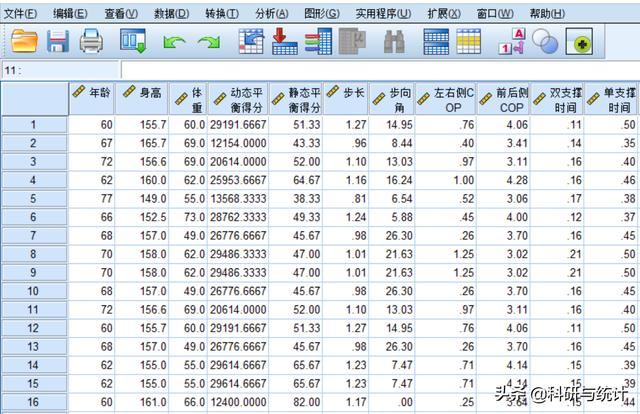图11.SPSS步骤：1)分析-回归-线性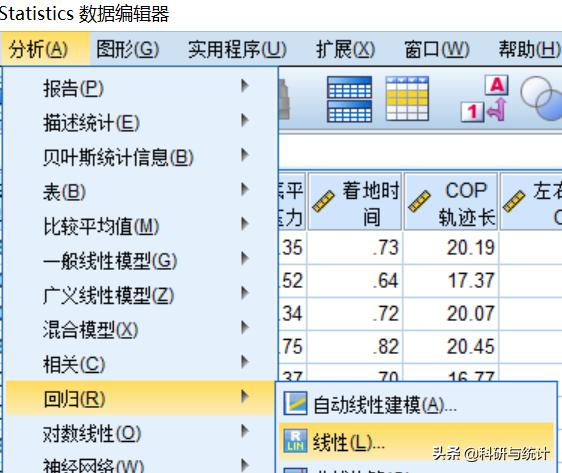图22)"动态平衡得分"选入"因变量"；"步长、步向角、左右测COP、前后侧COP、双支撑时间、单支撑时间"选入"自变量"。图33)按下图勾选。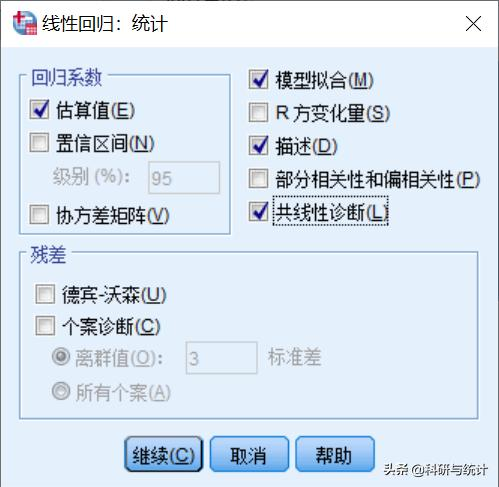图42.结果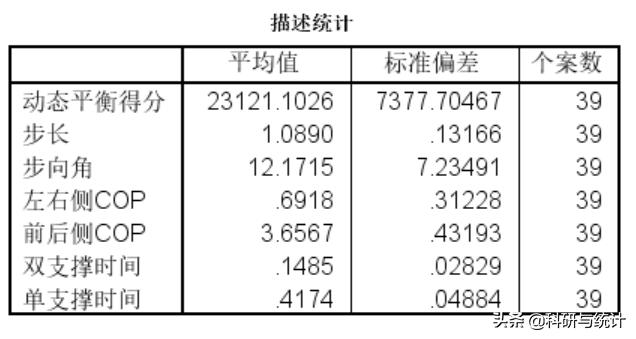图5图5给出了各指标的均值、标准差。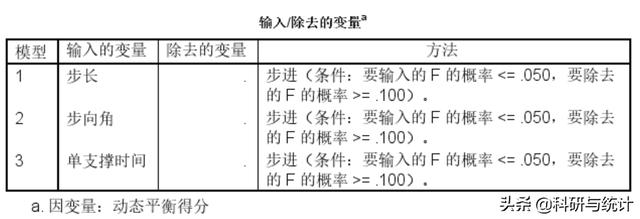图6图6，共建立3个回归模型，同时给出了依次输入的变量，由于引入新变量后，原来模型中引入的自变量仍然有统计学意义，因此没有自变量被除去。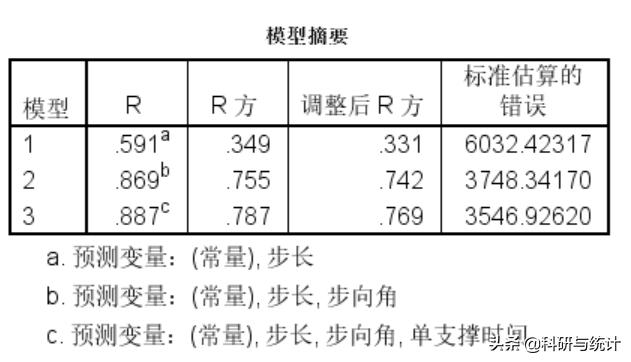图7(1)复相关系数R，表示模型中所有自变量与因变量之间的线性回归关系的密切程度。实际上是yi与估计值y^的简单线性回归系数。取值范围(0，1)。复相关系数在评价多重线性回归模型优劣时存在不足，即使向模型中增加的变量没有统计学意义，R值仍会增大。R值多大合适？不同的学科有不同的判断标准。社会科学类研究一般要求大于0.4，自然科学一般要求大于0.8。(2)决定系数R2，表示因变量y的总变异可由回归模型中自变量解释的部分所占比例。(3)调整后的决定系数R2adj。即使向模型中增加的变量没有统计学意义，R2值仍会增大，因此需要对其调整，形成调整后的决定系数R2adj。当模型中增加没有统计学意义的变量时，R2adj会变小，因此，R2adj是衡量模型好坏的重要指标之一。R2adj越大，模型的效果越好。案例模型的R2adj=0.768，模型效果较好。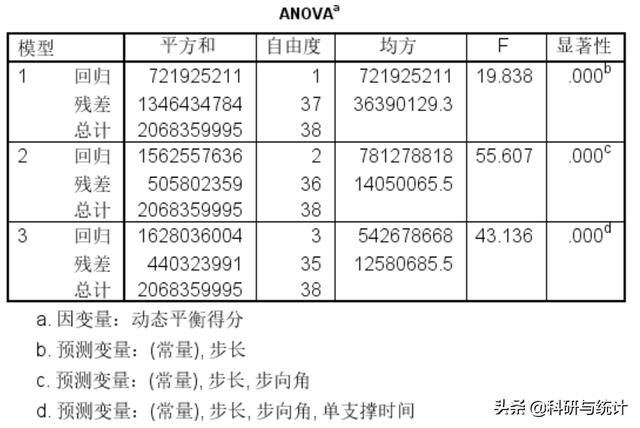图8回归模型的方差分析结果显示，三个模型的P值均小于0.05，说明回归模型有统计学意义。剩余标准差是误差均方的算术平方根，反映建立的模型预测因变量的精度。剩余标准差越小，模型的预测效果就越好。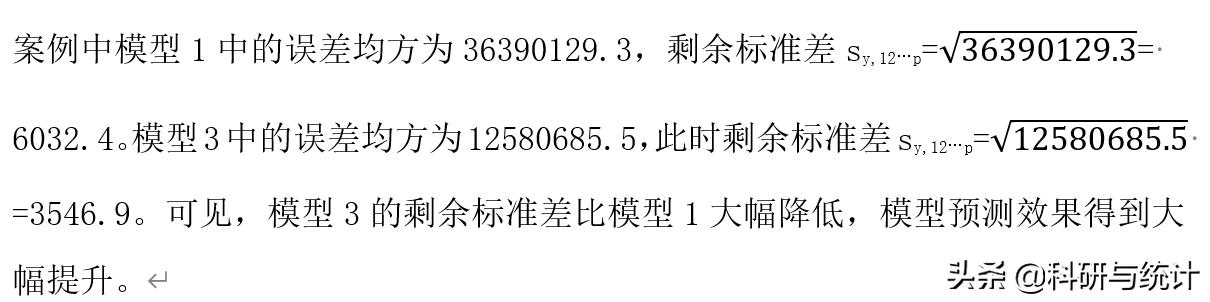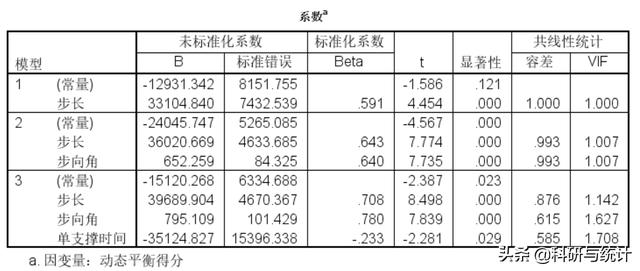图9给出了各模型自变量的偏回归系数估计值、标准化后的偏回归系数，以及回归系数的显著性检验结果。回归方程：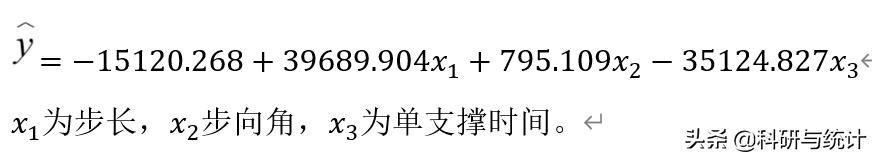标准化偏回归系数：由于不同自变量的偏回归系数的量纲不同，需要比较各自变量对因变量影响程度时，无法根据偏回归系数值的大小直接进行比较。标准化偏回归系数是偏回归系数标准化的结果，排除了量纲和自变量离散程度差异的影响，可以根据绝对值的大小判断自变量的重要程度。标准化偏回归系数分别为0.780，0.708，-0.233。多重共线性。多重共线性指自变量间存在线性相关关系，也就是一个自变量可以用其他一个或几个自变量的线性表达式进行表示。存在多重共线性时，模型可能会有以下表现：(1)模型检验结果为P≤α，但是各自变量的偏回归系数的检验结果却为P＞α。(2)专业上认为应该有统计学意义自变量检验结果却无统计学意义。(3)自变量的偏回归系数的取值大小甚至符号明显与实际情况相违背，难以解释。(4)增加或删除一个自变量或一个案例，自变量偏回归系数发生较大变化。以上情况使回归模型，特别是其中的回归系数难以有合乎专业知识的解释。要强调的是，多重共线性的存在不一定必然影响模型的使用价值。理论上，共线性不应当降低模型的预测效果，主要影响的是偏回归系数，导致模型无法用专业知识解释。多重共线性的识别(1)容忍度(tolerance)。某自变量的容忍度等于1减去以该自变量为因变量，以模型中其他自变量为自变量的线性回归模型的决定系数。容忍度越小，多重共线性就越严重。一般容忍度小于0.1时，认为存在严重的多重共线性。案例中的容忍度都偏大，说明不存在多重共线性(图9) 。(2)方差膨胀因子(VIF)，容忍度的倒数。VIF越大，多重共线性越严重。一般认为VIF要大于5或大于10时多重共线性较严重。案例中的方差膨胀因子都不大于2，进一步说明模型不存在多重共线性(图9) 。(3)特征值。对模型所有常数项及所有自变量提取主成分，如果自变量间存在较强的线性相关关系，则前面几个主成分特征值偏大，后面的主成分特征值偏小，甚至接近0。(4)条件指数。为最大主成分特征值与当前主成分特征值比值的算术平方根。所以第1个主成分的条件指数为1，如果条件指数偏大(如大于30)，则存在多重共线性。(5)变异构成。回归模型中各项(包括常数项)的变异能够被主成分解释的比例，或者说各主成分对模型中各项的贡献。如果某个主成分同时对两个或多个自变量的贡献都比较大，则说明这几个自变量间存在一定程度的共线性。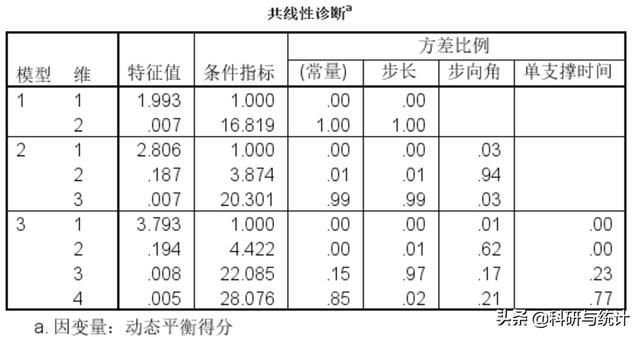多重共线性的处理(1)逐步回归法。只能在一定程度上解决多重共线性问题。(2)岭回归。能有效解决。(3)主成分回归。从存在多重共线性的自变量中提取主成分，然后将特征根较大的(如大于1)几个主成分与其他自变量一起进行多重线性回归，得出主成分回归系数，再根据主成分表达式反推出原始自变量的参数估计值。代价是提取主成分时丢失了一部分信息，收益是大大降低了共线性对参数估计值的扭曲，而且多重共线性越强，主成分丢失的信息越少，越适合做主成分回归。
展开全文• 提出了由回归模型和GM(1,1)模型构成灾害风险多模型预测方法,并建立了基于小误差概率—后验差比、相关系数—置信概率两种预测模型优选策略。根据5类风险记录体系和3种风险预警分析方法,建立了煤矿灾害风险预警...
• 简单线性回归模型用于分析1个自变量对1个因变量影响，或者说是由1个自变量预测1个因变量。但是事物间联系往往是多方面，因变量变化往往不是由单个自变量变化造成。探索多个自变量对1个因变量影响时，...
简单线性回归模型用于分析1个自变量对1个因变量的影响，或者说是由1个自变量预测1个因变量。但是事物间的联系往往是多方面的，因变量的变化往往不是由单个自变量的变化造成的。探索多个自变量对1个因变量的影响时，可以采用：多重线性回归分析。适用条件与简单线性回归分析类似，包括：线性趋势、独立性、正态性、方差齐性。但是对样本量有要求，样本量太少时，可能会出现检验效能不足，回归结果可靠性降低等问题。一般要求样本量要超过自变量数的20倍。案例：根据多个步态指标预测老年人动态平衡能力实验对象：60岁左右的老年人。测试内容：老年人平衡分数，以及步态指标。· 时间类指标：双支撑时间、单支撑时间。· 空间类指标：步长、步向角、左右侧COP、前后侧COP。研究目的：根据步态参数预测老年人平衡能力。本次分析目的：采用多个步态指标预测老年人的动态平衡能力部分数据：图11.SPSS步骤：1)分析-回归-线性图22)"动态平衡得分"选入"因变量"；"步长、步向角、左右测COP、前后侧COP、双支撑时间、单支撑时间"选入"自变量"。图33)按下图勾选。图42.结果图5图5给出了各指标的均值、标准差。图6图6，共建立3个回归模型，同时给出了依次输入的变量，由于引入新变量后，原来模型中引入的自变量仍然有统计学意义，因此没有自变量被除去。图7(1)复相关系数R，表示模型中所有自变量与因变量之间的线性回归关系的密切程度。实际上是yi与估计值y^的简单线性回归系数。取值范围(0，1)。复相关系数在评价多重线性回归模型优劣时存在不足，即使向模型中增加的变量没有统计学意义，R值仍会增大。R值多大合适？不同的学科有不同的判断标准。社会科学类研究一般要求大于0.4，自然科学一般要求大于0.8。(2)决定系数R2，表示因变量y的总变异可由回归模型中自变量解释的部分所占比例。(3)调整后的决定系数R2adj。即使向模型中增加的变量没有统计学意义，R2值仍会增大，因此需要对其调整，形成调整后的决定系数R2adj。当模型中增加没有统计学意义的变量时，R2adj会变小，因此，R2adj是衡量模型好坏的重要指标之一。R2adj越大，模型的效果越好。案例模型的R2adj=0.768，模型效果较好。图8回归模型的方差分析结果显示，三个模型的P值均小于0.05，说明回归模型有统计学意义。剩余标准差是误差均方的算术平方根，反映建立的模型预测因变量的精度。剩余标准差越小，模型的预测效果就越好。图9给出了各模型自变量的偏回归系数估计值、标准化后的偏回归系数，以及回归系数的显著性检验结果。回归方程：标准化偏回归系数：由于不同自变量的偏回归系数的量纲不同，需要比较各自变量对因变量影响程度时，无法根据偏回归系数值的大小直接进行比较。标准化偏回归系数是偏回归系数标准化的结果，排除了量纲和自变量离散程度差异的影响，可以根据绝对值的大小判断自变量的重要程度。标准化偏回归系数分别为0.780，0.708，-0.233。多重共线性。多重共线性指自变量间存在线性相关关系，也就是一个自变量可以用其他一个或几个自变量的线性表达式进行表示。存在多重共线性时，模型可能会有以下表现：(1)模型检验结果为P≤α，但是各自变量的偏回归系数的检验结果却为P＞α。(2)专业上认为应该有统计学意义自变量检验结果却无统计学意义。(3)自变量的偏回归系数的取值大小甚至符号明显与实际情况相违背，难以解释。(4)增加或删除一个自变量或一个案例，自变量偏回归系数发生较大变化。以上情况使回归模型，特别是其中的回归系数难以有合乎专业知识的解释。要强调的是，多重共线性的存在不一定必然影响模型的使用价值。理论上，共线性不应当降低模型的预测效果，主要影响的是偏回归系数，导致模型无法用专业知识解释。多重共线性的识别(1)容忍度(tolerance)。某自变量的容忍度等于1减去以该自变量为因变量，以模型中其他自变量为自变量的线性回归模型的决定系数。容忍度越小，多重共线性就越严重。一般容忍度小于0.1时，认为存在严重的多重共线性。案例中的容忍度都偏大，说明不存在多重共线性(图9) 。(2)方差膨胀因子(VIF)，容忍度的倒数。VIF越大，多重共线性越严重。一般认为VIF要大于5或大于10时多重共线性较严重。案例中的方差膨胀因子都不大于2，进一步说明模型不存在多重共线性(图9) 。(3)特征值。对模型所有常数项及所有自变量提取主成分，如果自变量间存在较强的线性相关关系，则前面几个主成分特征值偏大，后面的主成分特征值偏小，甚至接近0。(4)条件指数。为最大主成分特征值与当前主成分特征值比值的算术平方根。所以第1个主成分的条件指数为1，如果条件指数偏大(如大于30)，则存在多重共线性。(5)变异构成。回归模型中各项(包括常数项)的变异能够被主成分解释的比例，或者说各主成分对模型中各项的贡献。如果某个主成分同时对两个或多个自变量的贡献都比较大，则说明这几个自变量间存在一定程度的共线性。多重共线性的处理(1)逐步回归法。只能在一定程度上解决多重共线性问题。(2)岭回归。能有效解决。(3)主成分回归。从存在多重共线性的自变量中提取主成分，然后将特征根较大的(如大于1)几个主成分与其他自变量一起进行多重线性回归，得出主成分回归系数，再根据主成分表达式反推出原始自变量的参数估计值。代价是提取主成分时丢失了一部分信息，收益是大大降低了共线性对参数估计值的扭曲，而且多重共线性越强，主成分丢失的信息越少，越适合做主成分回归。
展开全文• 从20世纪70年代起，软件可靠性预测技术...它把在一定条件下出现事件按时间顺序加以排列，通过趋势外推数学模型预测未来。时间序列就是把统计资料按发生时间先后进行排列所得到一连串数字。时序分析是研究预测目
• 从20世纪70年代起，软件可靠性预测技术...它把在一定条件下出现事件按时间顺序加以排列，通过趋势外推数学模型预测未来。时间序列就是把统计资料按发生时间先后进行排列所得到一连串数字。时序分析是研究预测目
• 我们首先应用自回归综合移动平均（ARIMA）和广义自回归条件异方差（GARCH模型）来预测未来需求趋势和波动性，然后开发基于现代投资组合理论（MPT）方法来扩大DCN利用并套期保值。服务器过载风险。 进行了...
• 基于Jakes模型，开发了用于这种动态系统（即时变通道）回归模型（AR）。 更具体地说，我们提出增强均衡方法是将多级时域和频域均衡器与前馈环路结合在一起。 带下划线炒锅为均衡方法提供了一种统一...
• 利用多元GARCH模型估算了来自农村/地区，大城市，第三级和私人ICU的多个提供者系列的条件方差和相关性的时间动态。 对于平稳的第一阶差分序列，最适合的是t分布（自由度11.6）和ARMA（7,0）的t分布的非对称幂GARCH...
• Logistic 回归模型的损失函数为交叉熵，这类模型也叫对数线性模型，一般地，又叫做最大熵模型，这类模型和指数族分布的概率假设是一致的。对朴素贝叶斯假设，如果将其中的单元素的条件独立性做推广到一系列的隐变量...
条件随机场
我们知道，分类问题可以分为硬分类和软分类两种，其中硬分类有 SVM，PLA，LDA 等。软分类问题大体上可以分为概率生成和概率判别模型，其中较为有名的概率判别模型有 Logistic 回归，生成模型有朴素贝叶斯模型。Logistic 回归模型的损失函数为交叉熵，这类模型也叫对数线性模型，一般地，又叫做最大熵模型，这类模型和指数族分布的概率假设是一致的。对朴素贝叶斯假设，如果将其中的单元素的条件独立性做推广到一系列的隐变量，那么，由此得到的模型又被称为动态模型，比较有代表性的如 HMM，从概率意义上，HMM也可以看成是 GMM 在时序上面的推广。
我们看到，一般地，如果将最大熵模型和 HMM相结合，那么这种模型叫做最大熵 Markov 模型（MEMM）：

:root { --mermaid-font-family: "trebuchet ms", verdana, arial;}#mermaid-svg-J2e4JAOscz67xvYN {
color: rgba(0, 0, 0, 0.75);
font: ;
}x4y4x2y2x1y1x3y3
这个图就是将 HMM 的图中观测变量和隐变量的边方向反向，应用在分类中，隐变量就是输出的分类，这样 HMM 中的两个假设就不成立了，特别是观测之间不是完全独立的了。
HMM 是一种生成式模型，其建模对象为 $p(X,Y|\lambda)$，根据 HMM 的概率图，$p(X,Y|\lambda)=\prod\limits_{t=1}^Tp(x_t,y_t|\lambda,y_{t-1})$。我们看到，观测独立性假设是一个很强的假设，如果我们有一个文本样本，那么观测独立性假设就假定了所有的单词之间没有关联。
在 MEMM 中，建模对象是 $p(Y|X,\lambda)$，我们看概率图，给定 $y_t$，$x_t,x_{t-1}$ 是不独立的，这样，观测独立假设就不成立了。根据概率图，$p(Y|X,\lambda)=\prod\limits_{t=1}^Tp(y_t|y_{t-1},X,\lambda)$。
MEMM 的缺陷是其必须满足局域的概率归一化（Label Bias Problem），我们看到，在上面的概率图中，$p(y_t|y_{t-1},x_t)$， 这个概率，如果 $p(y_t|y_{t-1})$ 非常接近1，那么事实上，观测变量是什么就不会影响这个概率了。
对于这个问题，我们将 $y$ 之间的箭头转为直线转为无向图（线性链条件随机场），这样就只要满足全局归一化了（破坏齐次 Markov 假设）。

:root { --mermaid-font-family: "trebuchet ms", verdana, arial;}#mermaid-svg-EN9VOmNoCpgrmkRq {
color: rgba(0, 0, 0, 0.75);
font: ;
}x4y4x2y2x1y1x3y3
CRF 的 PDF
线性链的 CRF 的 PDF 为 $p(Y|X)=\frac{1}{Z}\exp\sum\limits_{t=1}^T(F_t(y_{t-1},y_t,x_{1:T}))$，两两形成了最大团，其中 $y_0$ 是随意外加的一个元素。作为第一个简化，我们假设每个团的势函数相同 $F_t=F$。
对于这个 $F$，我们进一步，可以将其写为 $F(y_{t-1},y_t,X)=\Delta_{y_{t-1},X}+\Delta_{y_{t},X}+\Delta_{y_t,y_{t-1},X}$这三个部分，分别表示状态函数已经转移函数，由于整体的求和，可以简化为 $F(y_{t-1},y_t,X)=\Delta_{y_{t},X}+\Delta_{y_t,y_{t-1},X}$。
我们可以设计一个表达式将其参数化：
KaTeX parse error: No such environment: align at position 8:
\begin{̲a̲l̲i̲g̲n̲}̲
\Delta_{y_t,y_…
其中 $g,f$ 叫做特征函数，对于 $y$ 有 $S$ 种元素，那么 $K\le S^2,L\le S$。
代入概率密度函数中：
$p(Y|X)=\frac{1}{Z}\exp\sum\limits_{t=1}^T[\sum\limits_{k=1}^K\lambda_kf_k(y_{t-1},y_t,X)+\sum\limits_{l=1}^L\eta_lg_l(y_t,X)]$
对于单个样本，将其写成向量的形式。定义 $y=(y_1,y_2,\cdots,y_T)^T,x=(x_1,x_2,\cdots,x_T)^T,\lambda=(\lambda_1,\lambda_2,\cdots,\lambda_K)^T,\eta=(\eta_1,\eta_2,\cdots,\eta_L)^T$。并且有 $f=(f_1,f_2,\cdots,f_K)^T,g=(g_1,g_2,\cdots,g_L)^T$。于是：
$p(Y=y|X=x)=\frac{1}{Z}\exp\sum\limits_{t=1}^T[\lambda^Tf(y_{t-1},y_t,x)+\eta^Tg(y_t,x)]$
不妨记：$\theta=(\lambda,\eta)^T,H=(\sum\limits_{t=1}^Tf,\sum\limits_{t=1}^Tg)^T$：
$p(Y=y|X=x)=\frac{1}{Z(x,\theta)}\exp[\theta^TH(y_t,y_{t-1},x)]$
上面这个式子是一个指数族分布，于是 $Z$ 是配分函数。
CRF 需要解决下面几个问题：

Learning：参数估计问题，对 $N$ 个 $T$ 维样本，$\hat{\theta}=\mathop{argmax}\limits_{\theta}\prod\limits_{i=1}^Np(y^i|x^i)$，这里用上标表示样本的编号。

Inference：

边缘概率：
$p(y_t|x)$

条件概率：一般在生成模型中较为关注，CRF 中不关注

MAP 推断：
$\hat{y}=\mathop{argmax}p(y|x)$

边缘概率
边缘概率这个问题描述为，根据学习任务得到的参数，给定了 $p(Y=y|X=x)$，求解 $p(y_t=i|x)$。根据无向图可以给出：
$p(y_t=i|x)=\sum\limits_{y_{1:t-1},y_{t+1:T}}p(y|x)=\sum\limits_{y_{1:t-1}}\sum\limits_{y_{t+1:T}}\frac{1}{Z}\prod\limits_{t'=1}^T\phi_{t'}(y_{t'-1},y_{t'},x)$
我们看到上面的式子，直接计算的复杂度很高，这是由于求和的复杂度在 $O(S^T)$，求积的复杂度在 $O(T)$，所以整体复杂度为 $O(TS^T)$。我们需要调整求和符号的顺序，从而降低复杂度。
首先，将两个求和分为：
KaTeX parse error: No such environment: align at position 8:
\begin{̲a̲l̲i̲g̲n̲}̲&p(y_t=i|x)=\fr…
对于 $\Delta_l$，从左向右，一步一步将 $y_t$ 消掉：
$\Delta_l=\sum\limits_{y_{t-1}}\phi_t(y_{t-1},y_t=i,x)\sum\limits_{y_{t-2}}\phi_{t-1}(y_{t-2},y_{t-1},x)\cdots\sum\limits_{y_0}\phi_1(y_0,y_1,x)$
引入：
$\alpha_t(i)=\Delta_l$
于是：
$\alpha_{t}(i)=\sum\limits_{j\in S}\phi_t(y_{t-1}=j,y_t=i,x)\alpha_{t-1}(j)$
这样我们得到了一个递推式。
类似地，$\Delta_r=\beta_t(i)=\sum\limits_{j\in S}\phi_{t+1}(y_t=i,y_{t+1}=j,x)\beta_{t+1}(j)$。这个方法和 HMM 中的前向后向算法类似，就是概率图模型中精确推断的变量消除算法（信念传播）。
参数估计
在进行各种类型的推断之前，还需要对参数进行学习：
KaTeX parse error: No such environment: align at position 8:
\begin{̲a̲l̲i̲g̲n̲}̲\hat{\theta}&=\…
上面的式子中，第一项是对数配分函数，根据指数族分布的结论：
$\nabla_\lambda(\log Z(x^i,\lambda,\eta))=\mathbb{E}_{p(y^i|x^i)}[\sum\limits_{t=1}^Tf(y_{t-1},y_t,x^i)]$
其中，和 $\eta$ 相关的项相当于一个常数。求解这个期望值：
$\mathbb{E}_{p(y^i|x^i)}[\sum\limits_{t=1}^Tf(y_{t-1},y_t,x^i)]=\sum\limits_{y}p(y|x^i)\sum\limits_{t=1}^Tf(y_{t-1},y_t,x^i)$
第一个求和号的复杂度为 $O(S^T)$，重新排列求和符号：
KaTeX parse error: No such environment: align at position 8:
\begin{̲a̲l̲i̲g̲n̲}̲\mathbb{E}_{p(y…
和上面的边缘概率类似，也可以通过前向后向算法得到上面式子中的边缘概率。
于是：
$\nabla_\lambda L=\sum\limits_{i=1}^N\sum\limits_{t=1}^T[f(y_{t-1},y_t,x^i)-\sum\limits_{y_{t-1}}\sum\limits_{y_t}p(y_{t-1},y_t|x^i)f(y_{t-1},y_t,x^i)]$
利用梯度上升算法可以求解。对于 $\eta$ 也是类似的过程。
译码
译码问题和 HMM 中的 Viterbi 算法类似，同样采样动态规划的思想一层一层求解最大值。


展开全文• 摘要：利用MATLAB工具箱，以平均气温、日照时数、平均风速为输入变量，建立了新疆石河子地区棉花耗水量的RBF人工神经网络预测系统，通过2008年实测数据的检验表明，此预测系统网络模型的绝对误差最大为0.0967mm/d、...matlab
• 摘要：利用MATLAB工具箱，以平均气温、日照时数、平均风速为输入变量，建立了新疆石河子地区棉花耗水量的RBF人工神经网络预测系统，通过2008年实测数据的检验表明，此预测系统网络模型的绝对误差最大为0.0967mm/d、...matlab
• GARCH 模型(DynamicConditional Corelational Autoregressive Conditional Heteroscedasticity Model)，即动态相关多变量广义自回归条件异方差模型来度量两个或者多个不同时间序列数据的动态波动相关性。该模型放宽...
• 在多维SU分布基础上,采用动态条件相关性(DCC)和自回归条件密度技术,通过智能优化算法对条件高阶矩模型的时变参数进行估计.实证研究结果表明,多维条件高阶矩模型较好的拟合了收益率时间序列高阶矩动态特征,与之前的高...
• 第一个是资产定价条件偏态模型的渐近性质。我们从一个简单的基于消费的资产定价模型入手，将资产定价模型与分位数回归的正则性条件联系起来。在一定的假设条件下，我们证明了分位数回归估计是渐近一致的正态分布。 ...
• 基于Spark的向量自回归模型和结构向量自回归模型的设计与实现，李涛，张勖，向量自回归模型（VAR）是一种常用的计量经济模型，它是用来估计在没有任何先验约束的条件下内生变量之间动态关系的模型。向量自回
• 将所建议框架的结果与基于GARCH模型的波动预测的发现进行比较，该模型在有无结构性波动的情况下进行。 基于对S＆P 500，FTSE 100，SZSE Composite和FBMKLCI指数的分析，我们的发现表明，相对于具有和不具有波动性...
• 针对上述缺陷,提出了广义约束的增量学习支持向量机回归模型的动态质量建模方法,利用KKT条件及时淘汰对后续训练影响不大的样本,同时保留了含有重要信息的样本。以带钢热镀锌生产中锌层质量模型为研究对象,建立生产...
• 本文将改进的乔列斯基分解和惩罚函数等非参数方法应用到DCC模型的估计中，提出了非参数DCC模型（NPDCC）.NPDCC模型首先通过改进的乔列斯基分解方法，将DCC模型估计中复杂的协方差阵估计问题转化为一系列的回归模型，...
• §4.2 回归变量选择与逐步回归 §4.3 多因变量多元线性回归 §4.4 多因变量逐步回归 §4.5 双重筛选逐步回归 习题四 第五章 判别分析 §5.1 距离判别 §5.2 贝叶斯(Bayes)判别法及广义平方距离判别法 §5.3 ...
• 8.2.2 VAR(1)模型的平稳性条件和矩 339 8.2.3 向量AR(p)模型 340 8.2.4 建立一个VAR(p)模型 342 8.2.5 脉冲响应函数 349 8.3 向量滑动平均模型 354 8.4 向量ARMA模型 357 8.5 单位根非平稳性与协整 362 8.6 ...时间序列
• 12.3数学模型的MATLAB相关函数 12.3.1传递模型的函数 12.3.2零极点模型函数 12.3.3状态空间模型函数 第13章径向基网络算法分析与实现 13.1径向基网络模型 13.2径向基网络的学习算法 13.3广义回归神经网络 ...
• 隐马尔科夫模型（Hidden Markov Model，HMM），和回归、分类那些处理相互独立样本数据的模型不同，它用于...本实验在隐马尔科夫条件下实现Viterbi算法，包含实验报告和维特比动态图，帮助大家更清晰理解HMM模型
• 最后介绍了动态模型的模拟。《高等学校本科生公共课教材•统计模拟及其R实现》对每一章节中的例子，都给出了用R语言编写的模拟程序。 目录 第1章 预备知识 1.1 矩母函数与生成函数 1.2 条件期望和条件方差 1.3 随机...
• 正则化（regularization）是用于抑制过拟合的方法的统称，它通过动态调整估计参数的取值来降低模型的复杂度，以偏差的增加为代价来换取方差的下降。 在线性回归里，最常见的正则化方式就是在损失函数（loss function...机器学习
• 文章目录软件测试基础什么是软件测试发展现状职业发展测试流程软件测试分类黑盒测试和白盒测试静态测试和动态测试功能测试和性能测试功能测试性能测试回归测试、冒烟测试、随机测试回归测试冒烟测试随机测试单元测试...软件测试 单元测试 编程语言